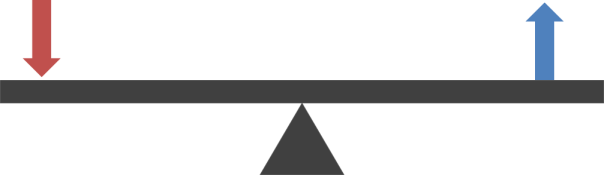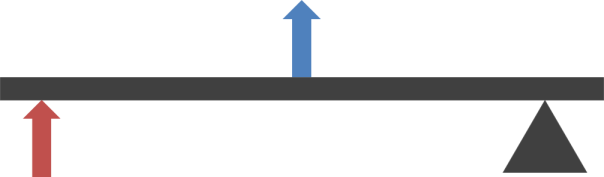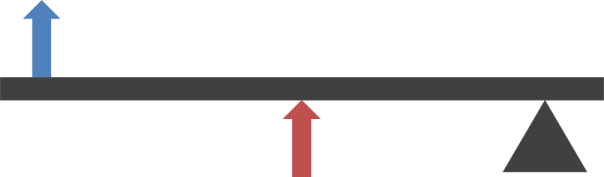# Lever Classes

The lever is one of the six simple machines.* It takes an input force and multiples it (by a factor known as the mechanical advantage) to create a larger output force.

Levers do not violate the conservation of energy because the input force has to be applied over a longer distance than the output force is applied over. (For example, a five newton force applied over one metre can produce a five hundred newton force over one centimetre; in both cases the work done is five joules.)

The operation of a lever is accomplished through the use of a rigid bar (the lever) and a fulcrum, or pivot. All levers can be placed into one of three classes depending of where the input force (red in the diagrams below), and output force (blue in the diagrams below) are applied relative to the fulcrum.

## Class 1

Examples: crowbar, scissors, pliersA class one lever is the type most familiar to people, and is often compared to a seesaw. The output force and the fulcrum are usually very close together, giving a very large mechanical advantage, as in the case of a crowbar, which typically has a mechanical advantage of six or more.

## Class 2

Examples: wheelbarrow, nutcrackers, bottle openerClass 2 levers are less familiar; many people using  a class 2 lever will not have realised that are using a lever at all. A class 2 lever typically has a mechanical advantage of two or three.

## Class 3

Examples: tweezers, tongs, human jawClass 3 levers have a mechanical advantage of less than one, meaning that the output force is lower than the input force, and they are therefore not very common. They are only used when a force is required to be applied at a distance, as in the case of barbeque tongs or tweezers; or when using a class two lever would be impossible, as in the case of the human jaw (the jaw muscles would have to be in front of the teeth).

* The other five simple machines are the wheel & axle, the pulley, the inclined plane (i.e. the ramp), the wedge, and the screw.

## 3 thoughts on “Lever Classes”

1. Anonymous says:

thank you kundi dahil sau d k marealise

2. Anonymous says:

Yo, fam yu web place is lit XD. Yo ma homies be like kkkk cuz fire in the crib dawg. Peace